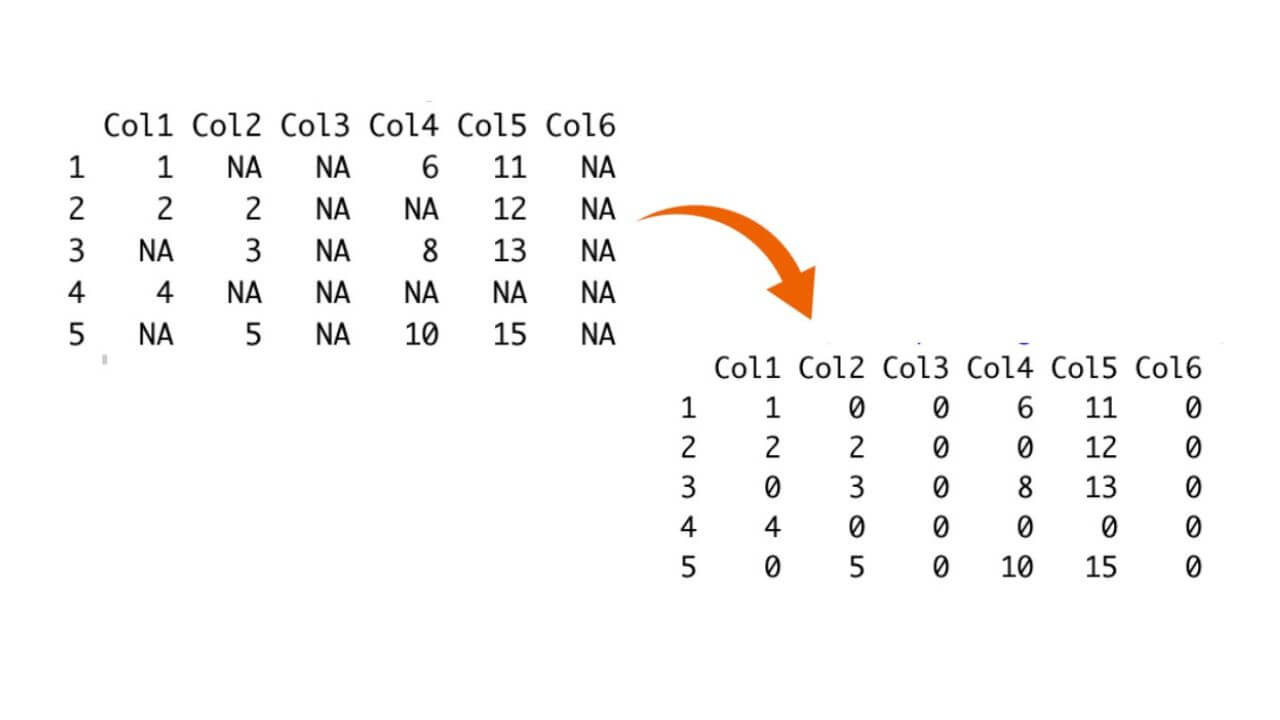# Replace NA with 0 (zero) in R | 5 Methods (with code)

Missing data is a common issue when working with datasets. In many cases, dealing with missing values is a critical step in data preprocessing, as it can significantly impact the results of your analysis. One approach to handling missing data is to replace NA (Not Available) values with 0s. In this article, we will explore various methods and techniques for replacing NAs with 0s in the R programming language.

## Understanding Missing Data in R

Before we dive into the methods for replacing NAs with 0s, let's first understand why missing data is a concern and why choosing the right strategy is crucial.

Missing data can arise for various reasons, such as equipment failures, human errors in data entry, or simply because certain data points are not applicable to some observations. Ignoring missing data or improperly handling it can lead to biased results, reduced statistical power, and incorrect conclusions in your analyses.

R represents missing data with the special value `NA`, which stands for "Not Available." It is essential to address NAs appropriately to ensure the integrity of your data analysis.

## Different Methods to Replace NA with 0s

R provides various different methods for us to replace NAs present in the dataset with 0s, all have some different use cases and can be used in different scenarios depending on our needs.Let us discuss them one by one.

### Method 1: Using the is.na() Function

One straightforward method to replace NAs with 0s in R is by using the is.na() function in combination with indexing. Here's how you can do it:

```# Create a sample vector with NAs
data <- c(1, 2, NA, 4, NA, 6)
```
```# Replace NAs with 0s
data[is.na(data)] <- 0
```

The ``is.na(data)`` function used in this example returns a logical vector containing TRUE for NAs and FALSE for non-missing values inside the vector. This logical vector is then used to index the data vector, and all the NAs are replaced with 0s.

### Method 2: Using the replace() Function

The replace() function is used for replacing NAs with zeros in R. You can employ this function to swap out values in a vector when a specific condition is met. Let's see how we can apply it to address NA replacements:

```# Create a sample vector with NAs
data <- c(1, 2, NA, 4, NA, 6)

# Replace NAs with 0s using replace()
data <- replace(data, is.na(data), 0)
```

The ``replace()`` function takes three arguments: the input vector, the condition (in this case, ``is.na(data)``), and the value to replace with (0).

### Method 3: Using the na.replace function from dplyr

If you are working with data frames, the `dplyr` package provides a convenient function called na.replace() to replace NAs with specific values. Here's how we can use it:

```library(dplyr)

df <- data.frame(A = c(1, 2, NA, 4, NA, 6),
B = c(NA, 2, 3, NA, 5, 6))

df <- df %>% na.replace(0)
```

The ``na.replace()`` function from `dplyr` replaces NAs in all columns of the data frame with the specified value (0 in this case)

### Method 4: Using the complete.cases() Function

In some cases, you may want to replace NAs only in specific columns or rows. To achieve this, you can use the complete.cases() function to identify rows with missing data and then replace NAs in those rows with 0s. Here's an example:

```df <- data.frame(
A = c(1, 2, NA, 4, NA),
B = c(NA, 2, 3, NA, 5),
C = c(6, 7, NA, 9, NA)
)

missing_rows <- !complete.cases(df\$A, df\$B)

df\$A[missing_rows] <- 0
df\$B[missing_rows] <- 0
```

In this example, we first use the ``complete.cases()`` function to identify rows with missing values in the data frame. Then, we use indexing to replace NAs in specific rows (A and B) with 0s.

### Method 5: Using the zoo Package

The zoo package provides advanced tools for handling time series data, including the ability to replace NAs efficiently. Here's how you can use the zoo package to replace NAs with 0s in a vector:

```library(zoo)

data <- c(1, 2, NA, 4, NA, 6)

data <- zoo::na.locf(data, na.rm = FALSE, fromLast = FALSE, zero = 0)
```

The ``na.locf()`` function from the zoo package replaces NAs with the last non-NA value in the vector, effectively filling in the missing values with 0s.

## Conclusion

Replacing NAs with 0s in R is a common data preprocessing task that ensures missing data does not adversely affect your analyses. In this article, we explored various methods and techniques for accomplishing this task, ranging from basic indexing and logical conditions to more advanced approaches using packages like `dplyr` and `zoo`. Remember that the choice of method depends on your specific dataset and analysis needs.

### FavTutor - 24x7 Live Coding Help from Expert Tutors!